# Introduction

This is the R-package accompanying the paper (Convex optimization for the densest subgraph and densest submatrix problems.

The problem of identifying a dense submatrix is a fundamental problem in the analysis of matrix structure and complex networks. This package provides tools for identifying the densest submatrix of a given graph using first-order optimization methods.

See the tutorials below to get started.

# The densest submatrix problem

Let $$[M] = \{1,2,\dots, M\}$$ for each positive integer $$M$$. Given a matrix $$\mathbf{A} \in R^{M\times N}$$, the densest $$m\times n$$-submatrix problem seeks subsets $$\bar U \subseteq {[M]}$$ and $$\bar V \subseteq {[N]}$$ of cardinality $$|\bar U|=m$$ and $$|\bar V| = n$$, respectively, such that the submatrix $$\mathbf{A}{[\bar U, \bar V]}$$ with rows index by $$\bar U$$ and columns indexed by $$\bar V$$ contains the maximum number of nonzero entries. That is, the densest $$m\times n$$-submatrix problem seeks the densest $$m\times n$$-submatrix of $$\mathbf{A}$$.

The densest $$m\times n$$-submatrix problem can be formulated as:

$\begin{equation} \min_{\mathbf{X}, \mathbf{Y} \in { \{0,1\}}^{M\times N} } {\operatorname{Tr}(\mathbf{Y} \mathbf{e} \mathbf{e}^T): \mathrm{P}_{\Omega}(\mathbf{X}-\mathbf{Y}) = \mathbf{0}, \operatorname{Tr}(\mathbf{X} \mathbf{e} \mathbf{e}^T) = mn, \operatorname{rank}(\mathbf{X}) = 1 }, \end{equation}$

where

• $$\mathrm{P}_{\Omega}$$ is the projection onto the index set of zero entries of matrix $$\mathbf A$$;

• $$\operatorname{Tr}$$ is the matrix trace function;

• $$\Omega$$ is the index set of zero entries of matrix $$A$$;

• $$\mathbf{X}$$ is rank-one matrix with $$mn$$ nonzero entries;

• $$\mathbf{Y}$$ is used to count the number of disagreements between $$\mathbf A$$ and $$\mathbf X$$;

• $$\mathbf{e}$$ - all-ones vector.

Unfortunately, optimization problems involving rank and binary constraints are intractable in general.

Relaxing the rank constraint with a nuclear norm penalty term, $$\|\mathbf{X} \|_* = \sum_{i=1}^N \sigma_i(\mathbf{X})$$, i.e., the sum of the singular values of matrix, and the binary constraints with box constraints yields the convex problem:

\begin{align} \min \; & \|\mathbf{X} \|_* + \gamma \operatorname{Tr}(\mathbf{Y} \mathbf{e} \mathbf{e}^T) \\ \operatorname{s.t.}\; & \operatorname{Tr}(\mathbf{X} \mathbf{e} \mathbf{e}^T) = mn, \\ & \mathrm{P}_\Omega(\mathbf{X} - \mathbf{Y}) = \mathbf{0}, \\ & \mathbf{Y} \ge \mathbf{0}, \\ & \mathbf{0} \le \mathbf{X} \le \mathbf{e} \mathbf{e}^T, \end{align} where $$\gamma >0$$ is a regularization parameter chosen to tune between the two objectives.

It can be shown that the relaxation is exact when binary matrix $$\mathbf{A}$$ contains a single, relatively large dense $$m\times n$$ block. For more information, see (Convex optimization for the densest subgraph and densest submatrix problems

# Alternating Direction Method of multipliers for densest submatrix problem

The alternating direction method of multipliers (ADMM) has been succesfully used in a broad spectrum of applications. The ADMM solves convex optimization problems with composite objective functions subject to equality constraints.

We direct the reader to Prof. Stephen Boyd’s website (ADMM) for a more thorough discussion of the ADMM.

To apply ADMM to our problem, we introduce artificial variables $$\mathbf{Q}$$, $$\mathbf{W}$$ and $$\mathbf{Z}$$ to obtain the equivalent optimization problem:

\begin{align} \min \; & \|\mathbf{X} \|_* + \gamma \|\mathbf{Y} \|_1 +{1}_{\Omega_Q}(\mathbf{Q})+{1}_{\Omega_W}(\mathbf{W})+{1}_{\Omega_Z}(\mathbf{Z})\\ \operatorname{s.t.}\; & \mathbf{X}-\mathbf{Y}=\mathbf{Q},\mathbf{X}=\mathbf{W}, \mathbf{X}=\mathbf{Z} \end{align}

where

• $$\Omega_Q = \{\, \mathbf{Q}\in R^{M\times N} \mid P_{\tilde{N}}(Q)=0 \, \}$$,
• $$\Omega_W =\{\, \mathbf{W}\in R^{M\times N} \mid \mathbf{e}^T\mathbf{W} \mathbf{e}=mn \, \}$$,
• $$\Omega_Z =\{\, \mathbf{Z}\in R^{M\times N} \mid {Z}_{ij}\leq 1 \forall (i,j)\in M\times N \, \}$$.

Here $${1}_{S}: R^{M\times M} \rightarrow \left \{0,+\infty \right \}$$ is the indicator function of the set $$S \subseteq R^{M\times N}$$, such that $${1}_S(\mathbf{X})=0$$ if $$\mathbf{X}\in S$$, and $$+\infty$$ otherwise.

Since our objective function is separable, we iteratively solve this optimization program using the ADMM. The basic idea is to rotate through $$3$$ steps:

1. minimize the augmented Lagrangian over primal variables,
2. update dual variables usng the updated primal variables,
3. calculate primal and dual residuals.

Interested readers are referred to (Convex optimization for the densest subgraph and densest submatrix problems. We include a summary of the algorithm below.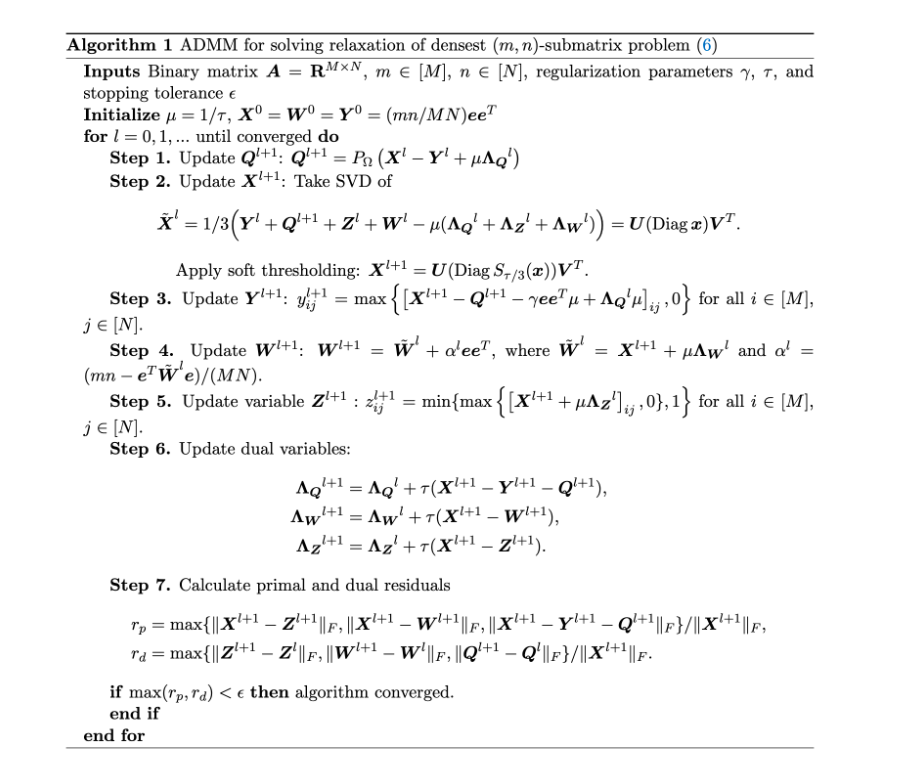# Examples

We test this package on two different types of data: first, using random matrices sampled from the planted dense $$m \times n$$ submtarix model and, second, real-world collaboration and communication networks.

## Random matrices

We first generate a random matrix with noise obscuring the planted submatrix using the function plantedsubmatrix. and then call the function densub to recover the planted submatrix.

# Initialize problem size and densities
# You can play around with these parameters
M=100 #number of rows of sampled matrix
N=200 #number of columns of sampled matrix
m=50 #number of rows of dense submatrix
n=40 #number of columns of dense submatrix
p=0.25 # noise density
q=0.85 #in-group density

#Make binary matrix with mn-submatrix
random<-plantedsubmatrix(M=M, N=N,m=m,n=n,p=p,q=q)

After generating the structure random containing the random matrix with desired planted structure, we can visually represent the matrix and planted submatrix as two-tone images, where dark pixels correspond to nonzero entries, and light pixels correspond to zero entries, using the following commands.


# Plot sampled G and matrix representations.
image(random$sampled_matrix, useRaster=TRUE, axes=FALSE, main = "Matrix A") image(random$dense_submatrix, useRaster=TRUE, axes=FALSE, main = "Matrix X0")
image(random$disagreements, useRaster=TRUE, axes=FALSE, main = "Matrix Y0") Tne vizualization of the randomly generated matrix $$\mathbf{A}$$ helps us to understand its structure. It is clear that $$\mathbf{A}$$ contains a dense $$50 \times 40$$ block (in the bottom left corner).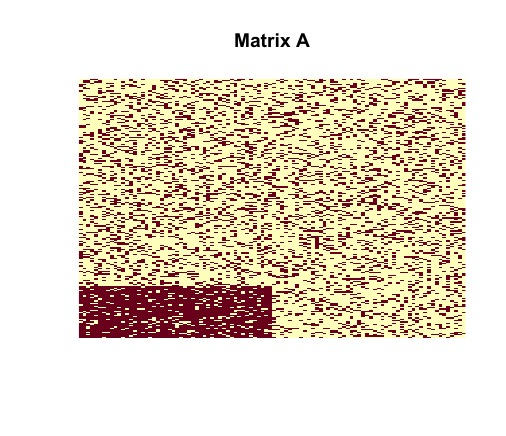Visual representation of randomly generated $$\mathbf{A}$$ We can remove all noise and isolate an image of a rank-one matrix $$\mathbf{X0}$$ with $$mn$$ nonzero entries.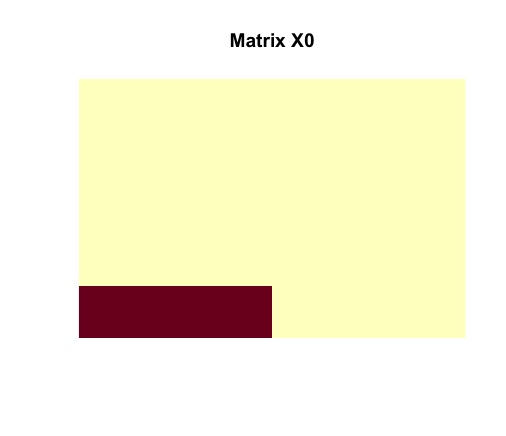Visual representation of dense submatrix Then we vizualize matrix $$\mathbf{Y0}$$ to see the number of disagreements between original matrix $$\mathbf{A}$$ and $$\mathbf{X0}$$.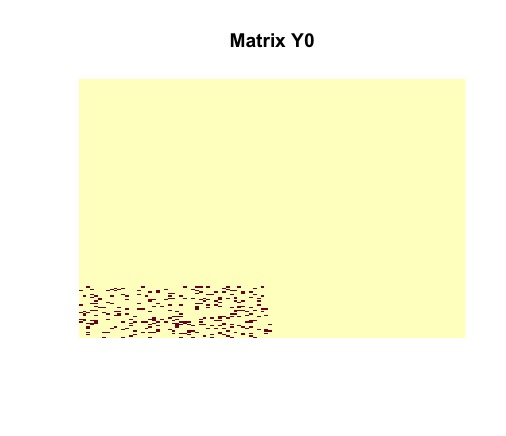Disagreement between $$\mathbf{A}$$ and $$\mathbf{X_0}$$ We call the ADMM solver and visualize the output using the following commands. #Call ADMM solver admm<-densub(G=random$sampled_matrix, m=m, n=n, tau = 0.35, gamma = 6/(sqrt(m*n)*(q-p)), opt_tol = 1.0e-4,maxiter=500, quiet = TRUE)

#Plot results
image(admm$X, useRaster=TRUE, axes=FALSE, main = "Matrix X") image(admm$Y, useRaster=TRUE, axes=FALSE, main = "Matrix Y")

The ADMM solver returns the optimal solutions $$\mathbf{X}$$ and $$\mathbf{Y}$$. It must be noted that matrices $$\mathbf X$$ and $$\mathbf Y$$ are identical to the actual structures of $$\mathbf{X_0}$$ and $$\mathbf{Y_0}$$. The planted submatrix is recovered.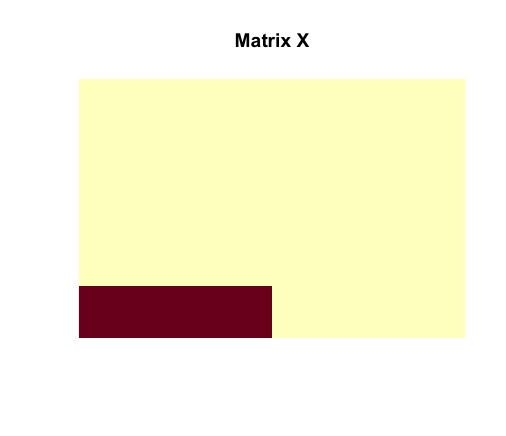Optimal solution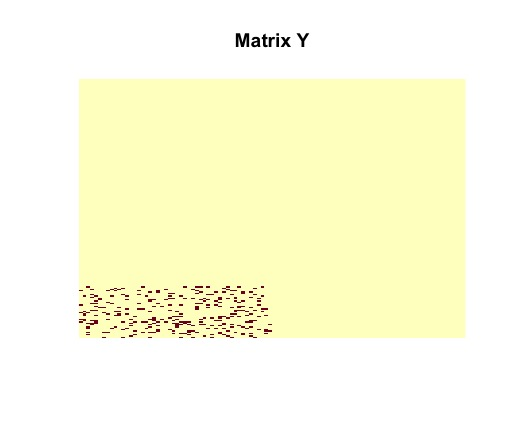Optimal Solution

## Collaboration Network

The following is a simple example on how one could use the package to analyze the collaboration network found in the JAZZ dataset. It is known that this network contains a cluster of $$100$$ musicians which performed together.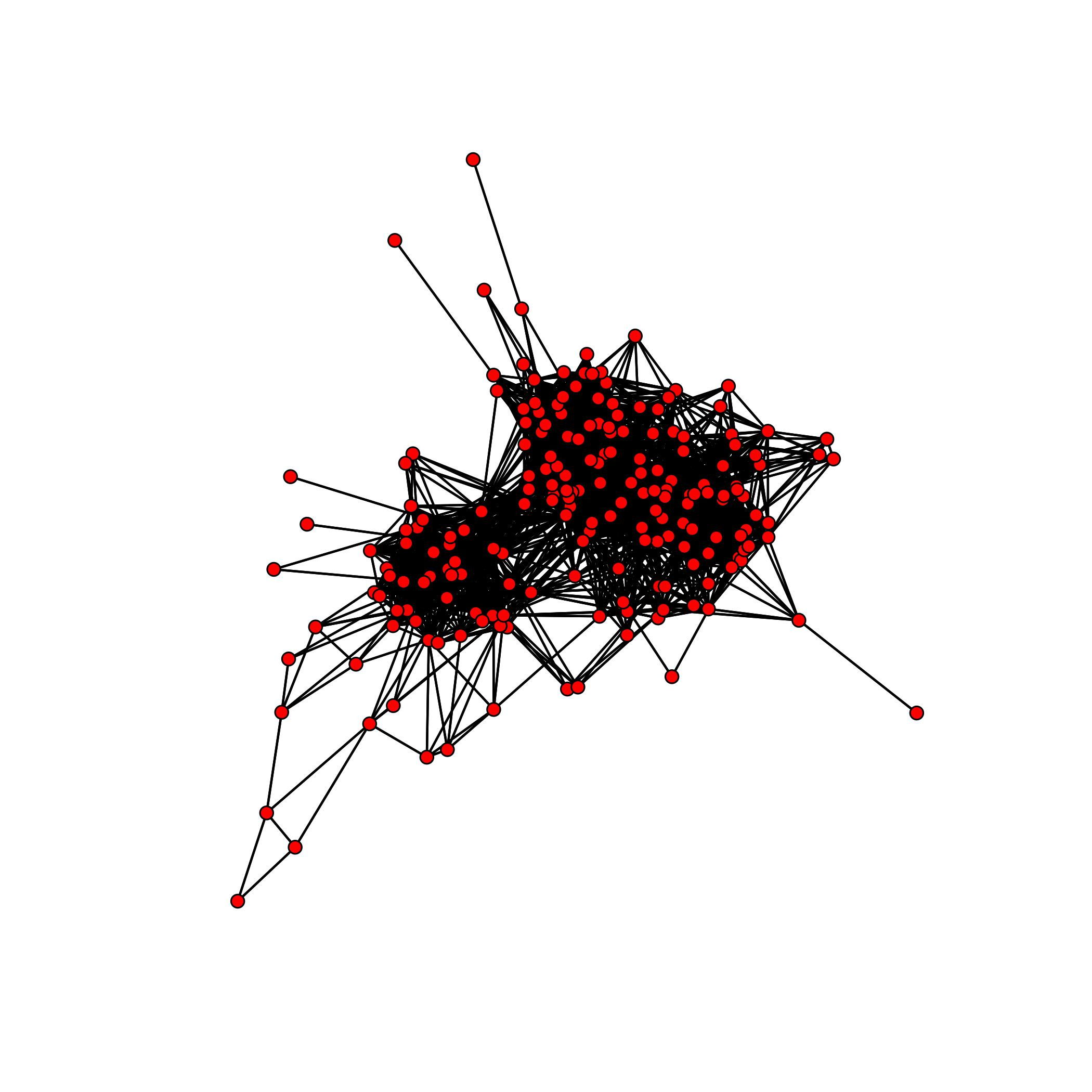JAZZ Network

We have already prepared dataset to work with. More details can be found in the provided file JAZZ_IN_R.R.

#Load dataset

#Initialize problem size and densities
G=new #define matrix G equivalent to JAZZ dataset
m=100 #clique size or the number of rows of the dense submatrix
n=100 #clique size of the number of columns of the dense sumbatrix
tau=0.85 #regularization parameter
opt_tol=1.0e-2 #optimal tolerance
verbose=1
maxiter=2000 #number of iterations
gamma=8/n #regularization parameter

admm <- densub(G = G, m = m, n = n, tau = tau, gamma = gamma, opt_tol = opt_tol, maxiter=maxiter, quiet = TRUE)
# Planted solution X0.
X0=matrix(0L, nrow=198, ncol=198) #construct rank-one matrix X0
X0[1:100,1:100]=matrix(1L, nrow=100, ncol=100)#define dense block

# Planted solution Y0.
Y0=matrix(0L, nrow=198, ncol=198) #construct matrix for counting disagreements between G and X0
Y0[1:100,1:100]=matrix(1L,nrow=100,ncol=1000)-G[1:100,1:100]

#Check primal and dual residuals.
}
Our algorithm converges to the dense submatrix representing the community of $$100$$ musicians after $$50$$ iterations.